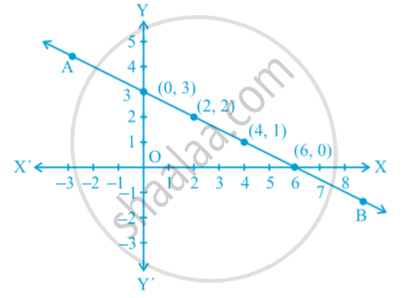# Graph of a Linear Equation in Two Variables

#### notes

The solutions of a linear equation in two variables algebraically.
Example : x +2y = 6      ...(1)
can be expressed in the form of a table as follows by writing the values of y below the corresponding values of x :

 X 0 2 4 6 ... Y 3 2 1 0 ...

Let us plot the points (0, 3), (2, 2), (4, 1) and (6, 0) on a graph paper. Now join any two of these points and obtain a line. Let us call this as line AB in following fig.Now, pick another point on this line, say (8, –1). Pick any other point on this line AB and verify whether its coordinates satisfy the equation or not.
1. Every point whose coordinates satisfy Equation (1) lies on the line AB.
2. Every point (a, b) on the line AB gives a solution x = a, y = b of Equation (1).
3. Any point, which does not lie on the line AB, is not a solution of Equation (1).
The every point on the line satisfies the equation of the line and every solution of the equation is a point on the line. In fact, a linear equation in two variables is represented geometrically by a line whose points make up the collection of solutions of the equation. This is called the graph of the linear equation. So, to obtain the graph of a linear equation in two variables, it is enough to plot two points corresponding to two solutions and join them by a line.

Remark :
The reason that a, degree one, polynomial equation ax + by + c = 0 is called a linear equation is that its geometrical representation is a straight line.

If you would like to contribute notes or other learning material, please submit them using the button below.

### Shaalaa.com

Graphs of linear equations in two variables. [00:14:53]
S
0%